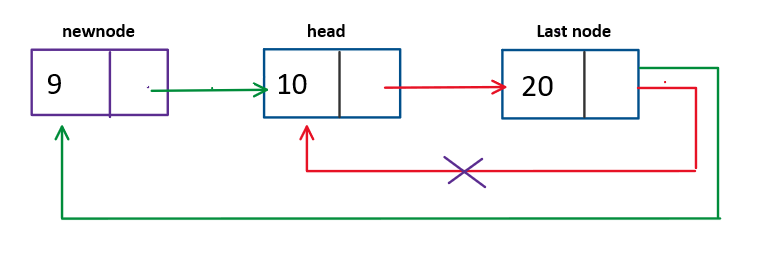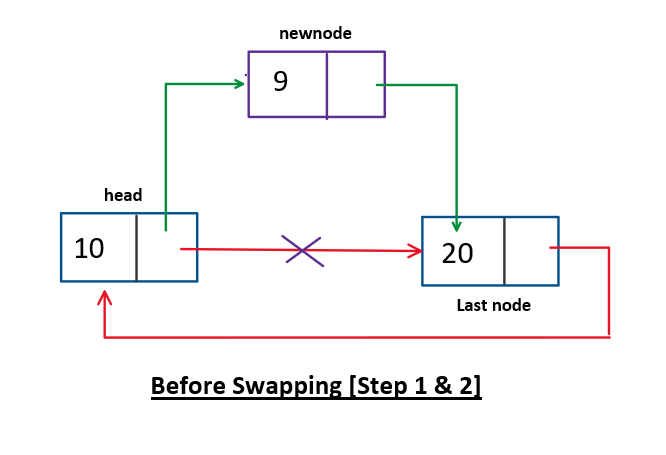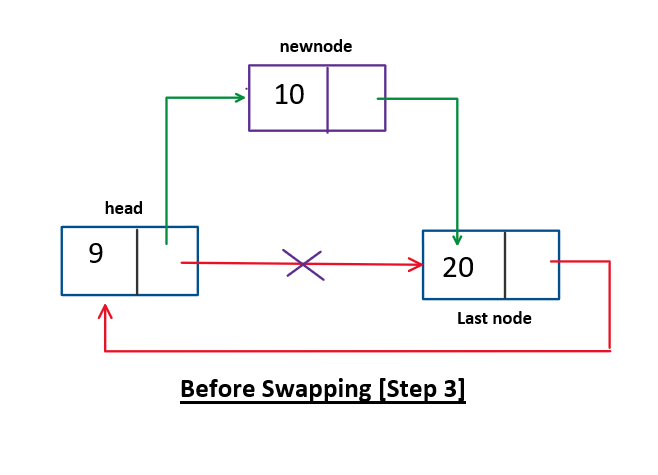# Insertion at begin of circular linked list

Problem Statement: Given a Circular Linked List, insert an element at begin of the circular linked list.

Examples:

```Example 1:
Input: 1 2 3 4 5 6, newNode=0
Output: 0 1 2 3 4 5 6

Example 2:
Input: 10, newNode=9
Output: 9 10

Example 3:
Input: NULL, newNode=1
Output: 1
```

### Solution:

DisclaimerDon’t jump directly to the solution, try it out yourself first.

### Solution 1:

Approach: O(n) approach

To achieve this, we iterate to the end of the CLL and perform the following operations

1. Make the next pointer of the last node to point to the newNode.
2. Make the next pointer of the newNode to point to the head node
3. Return the newNode as the head of our CLL.

The following diagram gives a simple visualizationCode:

## C++ Code

``````#include <iostream>
using namespace std;

struct node {
int data;
node * next;
node(int d) {
data = d;
next = NULL;
}
};
node * insertion_beg(node * head, int x) {
node * newnode = new node(x);
if (head == NULL) { // CLL is empty
newnode -> next = newnode;
return newnode;
} else {
//   O(n) approach
node * current = head;
while (current -> next != head) {
current = current -> next;
}
newnode -> next = current -> next;
current -> next = newnode;
return newnode;
}
}
//printing CLL
void printCLL(node * head) {
node * current = head;
do {
cout << current -> data << " ";
current = current -> next;
} while (current != head);
cout<<endl;
}
int main() {
node * head = new node(10);
head -> next = new node(20);
head -> next -> next = head;
cout<<"Before insertion:"<<endl;
cout<<"Before insertion:"<<endl;
return 0;
}
``````

Output:

Before insertion:
10 20
Before insertion:
9 10 20

Time complexity: O(n)

Space complexity: O(1)

## Java Code

``````class node {
int data;
node next;
node(int d) {
data = d;
next = null;
}
}
public class Main {
static node insert_begg(node head, int x) {
node newnode = new node(x);
if (head == null) {
newnode.next = newnode;
return newnode;
} else {
node current = head;
while (current.next != head) {
current = current.next;
}
current.next = newnode;
return newnode;
}
}
public static void printlist(node head) {
if (head == null) return;
node current = head;
do {
System.out.print(current.data + " ");
current = current.next;
} while (current != head);
System.out.println();
}
public static void main(String args[]) {
node head = new node(10);
head.next = new node(20);
head.next.next = new node(30);
System.out.println("Before insertion: ");
System.out.println("After insertion: ");
}
}
``````

Output:

Before insertion:
10 20 30
After insertion:
15 10 20 30

Time complexity: O(n)

Space complexity: O(1)

### Solution 2:

Approach: O(1) approach

This approach is very simple. Here we do the following steps:

1. Make the newNode as the second node of our CLL.
2. Swap the data of our head node and newNode
3. Return the head as our head node of our CLL

The following diagram backs the above approachCode:

## C++ Code

``````#include <iostream>
using namespace std;

struct node {
int data;
node * next;
node(int d) {
data = d;
next = NULL;
}
};
node * insertionBeg(node * head, int x) {
node * newnode = new node(x);
//step 1
newnode -> next = head -> next;
head -> next = newnode;

//step 2
swap(newnode -> data, head -> data);

//step 3
}
void printCLL(node * head) {
node * current = head;
do {
cout << current -> data << " ";
current = current -> next;
} while (current != head);
cout<<endl;
}
int main() {
node * head = new node(10);
head -> next = new node(20);
head -> next -> next = head;
cout<<"Before insertion:"<<endl;
cout<<"After insertion:"<<endl;
return 0;
}
``````

Output:

Before insertion:
10 20
After insertion:
9 10 20

Time Complexity: O(1)

Space Complexity: O(1)

## Java Code

``````class node {
int data;
node next;
node(int d) {
data = d;
next = null;
}
}
public class Main {
static node insert_begg(node head, int x) {
node newnode = new node(x);
if (head == null) {
newnode.next = newnode;
return newnode;
} else {
// step 1

//step 2
int swapper = head.data;
newnode.data = swapper;

//step 3
}
}
public static void printlist(node head) {
if (head == null) return;
node current = head;
do {
System.out.print(current.data + " ");
current = current.next;
} while (current != head);
System.out.println();
}
public static void main(String args[]) {
node head = new node(10);
head.next = new node(20);
head.next.next = new node(30);
System.out.println("Before insertion:");
System.out.println("After insertion:");
}
}
``````

Output:

Before insertion:
10 20 30
After insertion:
15 10 20 30

Time Complexity: O(1)

Space Complexity: O(1)

Special thanks to Yash Mishra for contributing to this article on takeUforward. If you also wish to share your knowledge with the takeUforward fam, please check out this article